## Example Questions

### Example Question #1 : Lowest Common Denominator

What is the least common denominator ofand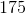?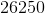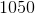Explanation:

To find the least common denominator of two numbers, it is easiest first to factor them into prime factors: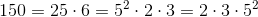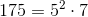Now, you need to compare each number and choose the case in which the prime factor has the highest power.  Therefore, sinceandare found only in, you will select those.  You can take "either"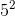.  Finally, theinis the largest factor of.  Your LCD is found by multiplying all of these together: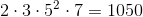### Example Question #1 : Lowest Common Denominator

Simplify: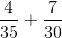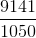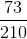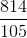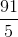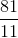Explanation:

To begin to solve this, you need to find the least common denominator ofand.  The easiest way to do this is to begin by factoring them into prime factors: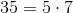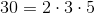The LCD is found by selecting the largest power for each factor across the two values.  Therefore, you will take,, andfromand thefrom the.  Your LCD is therefore: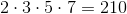.

Now, apply this to your fractions: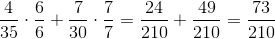### Example Question #1 : How To Find The Lowest / Least Common Denominator

In simplest form,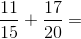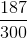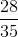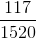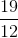Explanation:

First, find the smallest number that bothandwill factor into, which is.

This means that the fist fraction should be multiplied byand the second should be multiplied by.

Therefore,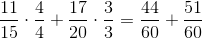.

Then, add the numerators while the denomenators stay the same: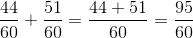.

Then, reduce the fraction to its lowest terms: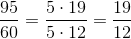.

### All GRE Math Resources